# IB DP Physics: HL复习笔记10.2.5 Escape Speed

### Escape Speed

• To escape a gravitational field, a mass must travel at the escape velocity
• This is dependent on the mass and radius of the object creating the gravitational field, such as a planet, a moon or a black hole
• Escape velocity is defined as:

The minimum speed that will allow an object to escape a gravitational field with no further energy input

• It is the same for all masses in the same gravitational field ie. the escape velocity of a rocket is the same as a tennis ball on Earth
• An object reaches escape velocity when all its kinetic energy has been transferred to gravitational potential energy
• This is calculated by equating the equations:
•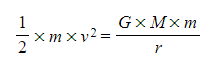Where:
• m = mass of the object in the gravitational field (kg)
• v = escape velocity of the object (m s-1)
• G = Newton's Gravitational Constant
• M = mass of the object to be escaped from (ie. a planet) (kg)
• r = distance from the centre of mass M (m)
• Since mass m is the same on both sides of the equations, it can cancel on both sides of the equation: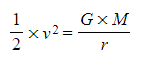• Multiplying both sides by 2 and taking the square root gives the equation for escape velocity, v: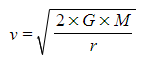• This equation is not given on the datasheet. Be sure to memorise how to derive it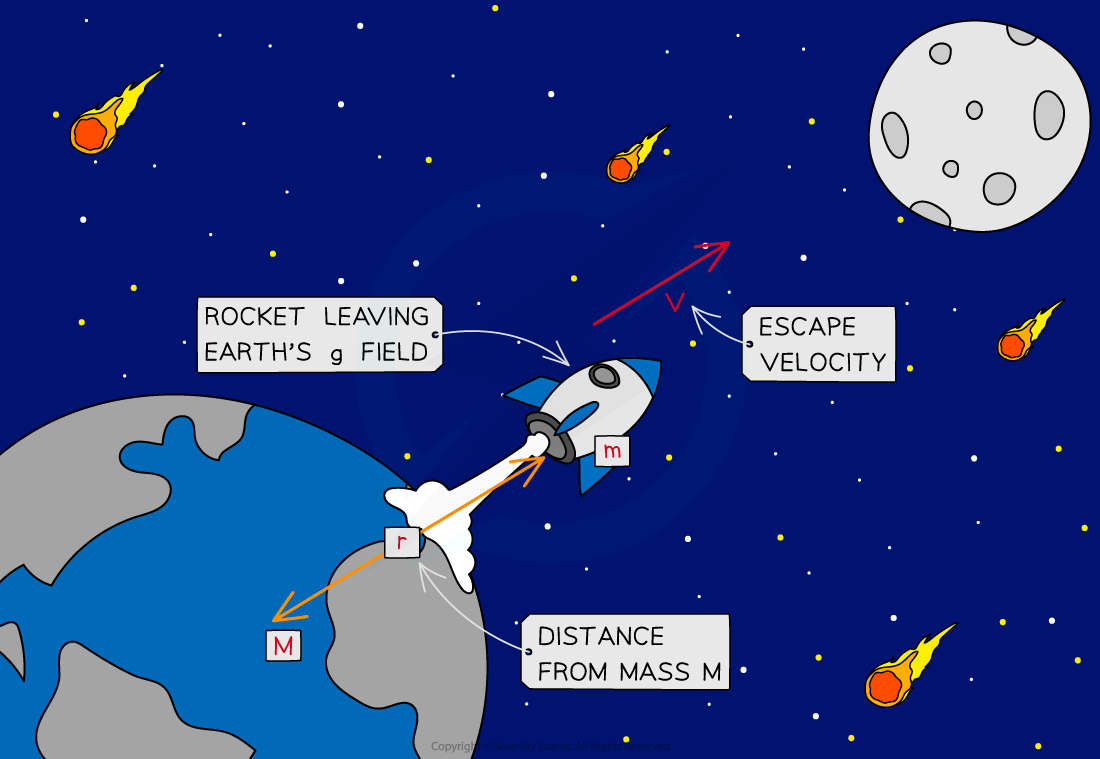For an object to leave the Earth's gravitational field, it will have to travel at a speed greater than the Earth's escape velocity, v

• Rockets launched from the Earth's surface do not need to achieve escape velocity to reach their orbit around the Earth
• This is because:
• They are continuously given energy through fuel and thrust to help them move
• Less energy is needed to achieve orbit than to escape from Earth's gravitational field
• The escape velocity is not the velocity needed to escape the planet but to escape the planet's gravitational field altogether
• This could be quite a large distance away from the planet

#### Worked Example

Calculate the escape velocity at the surface of the Moon given that its density is 3340 kg m-3 and has a mass of 7.35 × 1022 kg.Newton's Gravitational Constant = 6.67 × 10-11 N m2 kg-2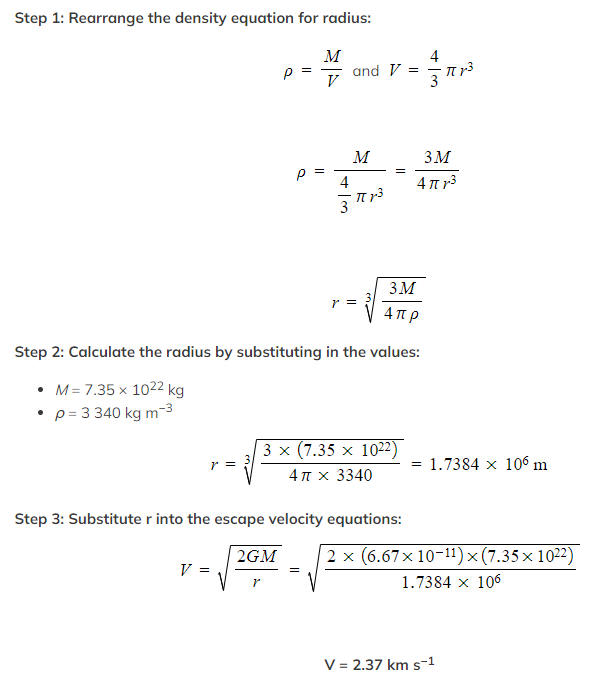Exam Tip

When writing the definition of escape velocity, avoid terms such as 'gravity' or the 'gravitational pull / attraction' of the planet. It is best to refer to its gravitational field.